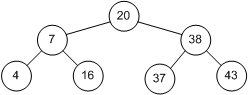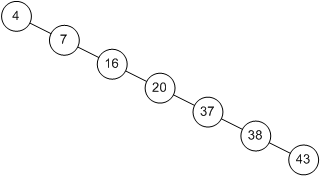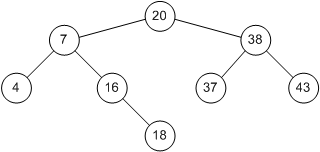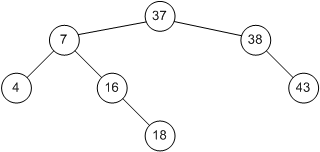# Binary Search Trees

In the introduction we used the binary search algorithm to find data stored in an array. This method is very effective, as each iteration reduced the number of items to search by one-half. However, since data was stored in an array, insertions and deletions were not efficient. Binary search trees store data in nodes that are linked in a tree-like fashion. For randomly inserted data, search time is O(lgn). Worst-case behavior occurs when ordered data is inserted. In this case the search time is O(n). See Cormen  for a more detailed description.

## Theory

A binary search tree is a tree where each node has a left and right child. Either child, or both children, may be missing. Figure 3-2 illustrates a binary search tree. Assuming krepresents the value of a given node, then a binary search tree also has the following property: all children to the left of the node have values smaller than k, and all children to the right of the node have values larger than k. The top of a tree is known as the root, and the exposed nodes at the bottom are known as leaves. In Figure 3-2, the root is node 20 and the leaves are nodes 4, 16, 37, and 43. The height of a tree is the length of the longest path from root to leaf. For this example the tree height is 2.Figure 3-2: A Binary Search Tree

To search a tree for a given value, we start at the root and work down. For example, to search for 16, we first note that 16 < 20 and we traverse to the left child. The second comparison finds that 16 > 7, so we traverse to the right child. On the third comparison, we succeed.

Each comparison results in reducing the number of items to inspect by one-half. In this respect, the algorithm is similar to a binary search on an array. However, this is true only if the tree is balanced. For example, Figure 3-3 shows another tree containing the same values. While it is a binary search tree, its behavior is more like that of a linked list, with search time increasing proportional to the number of elements stored.Figure 3-3: An Unbalanced Binary Search Tree

## Insertion and Deletion

Let us examine insertions in a binary search tree to determine the conditions that can cause an unbalanced tree. To insert an 18 in the tree in Figure 3-2, we first search for that number. This causes us to arrive at node 16 with nowhere to go. Since 18 > 16, we simply add node 18 to the right child of node 16 (Figure 3-4).

Now we can see how an unbalanced tree can occur. If the data is presented in an ascending sequence, each node will be added to the right of the previous node. This will create one long chain, or linked list. However, if data is presented for insertion in a random order, then a more balanced tree is possible.

Deletions are similar, but require that the binary search tree property be maintained. For example, if node 20 in Figure 3-4 is removed, it must be replaced by node 18 or node 37. Assuming we replace a node by its successor, the resulting tree is shown in Figure 3-5. The rationale for this choice is as follows. The successor for node 20 must be chosen such that all nodes to the right are larger. Therefore we need to select the smallest valued node to the right of node 20. To make the selection, chain once to the right (node 38), and then chain to the left until the last node is found (node 37). This is the successor for node 20.Figure 3-4: Binary Tree After Adding Node 18Figure 3-5: Binary Tree After Deleting Node 20

## Implementation in C

An ANSI-C implementation for a binary search tree is included. Typedefs `recType`, `keyType`, and comparison operators `compLT` and `compEQ` should be altered to reflect the data stored in the tree. Each Node consists of `left`, `right`, and `parent` pointers designating each child and the parent. The tree is based at`root`, and is initially `NULL`. Function `insert` allocates a new node and inserts it in the tree. Function `delete` deletes and frees a node from the tree. Function `find` searches the tree for a particular value.

## Implementation in Visual Basic

The binary tree algorithm has been implemented as objects, using a module for the algorithm, and a class for the nodes. It has also been implemented as a class, using arrays. The array implementation is recommended.